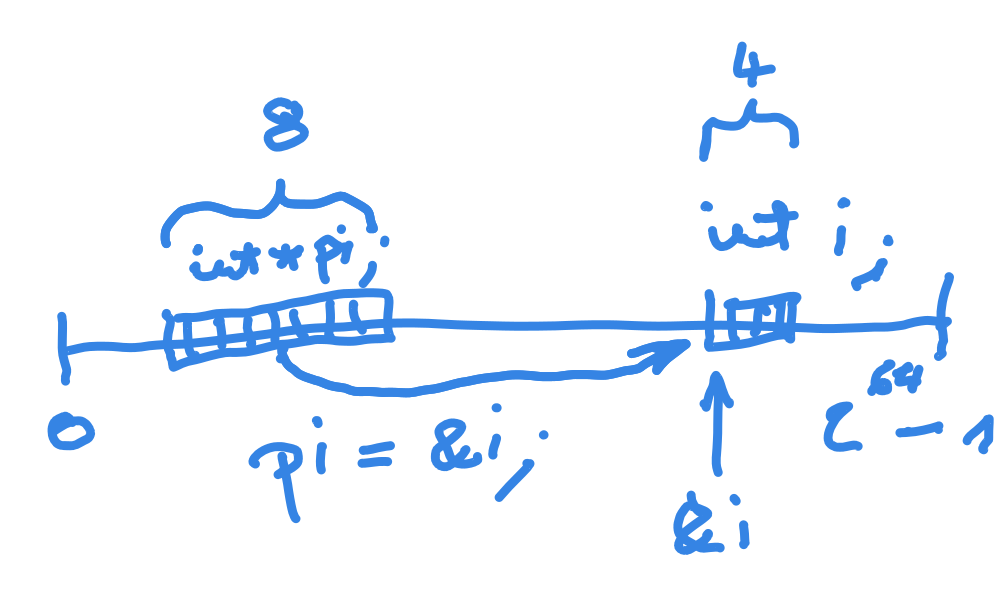## Pointers: Basics¶

• Variable that points to another variable

• Basis for e.g. call-by-reference

• Simple in theory

• Practically difficult and dangerous

Pointer to integer (64 bit)Showing the entire address space (0..2^64-1) (a Wacom sketch, made during a course)¶

## Pointer: Operators¶

Operations

• Taking an address: what is the address of the variable `i`?

• Dereferencing: what is the content of the memory location that a pointer points to?Taking an address ```int i = 35129; int *pi; pi = &i; ``` Dereferencing ```int value = *pi; /* value == 35129 */ ```

## More Examples¶

```int x = 1, y = 2;
int *pi; /* pointer to int */

pi = &x; /* "pi points to x" */
*pi == 1; /* true */
x = 42;
*pi == 42; /* true */
pi = &y;
*pi == 2; /* true */

*pi = *pi + 1;
*pi += 1;
y == 4; /* true */

pi = 0; /* null pointer */
```